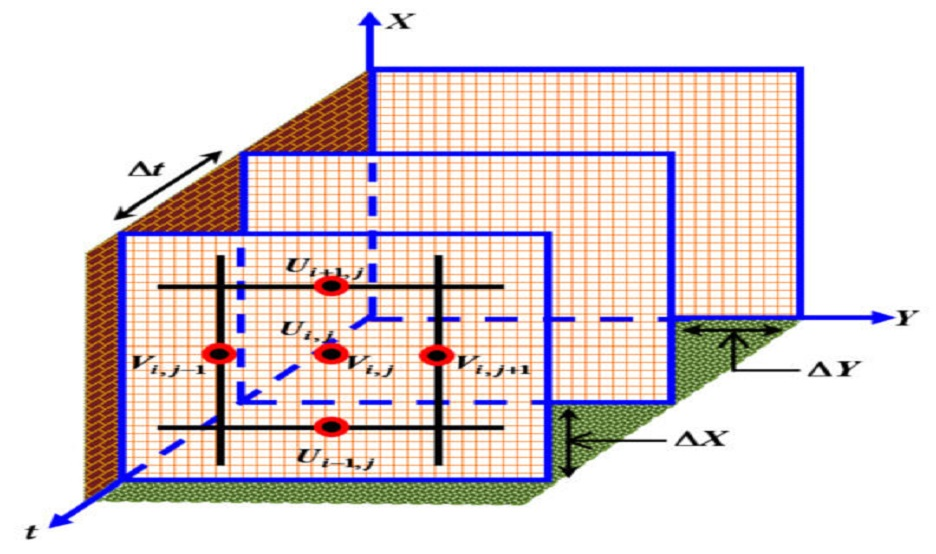#### Heat and Mass Transfer Analysis on Magneto Micropolar Fluid Flow with Heat Absorption in Induced Magnetic Field

Author:- Md. Mohidul Haque
Category:- Journal; Year:- 2021
Discipline:- Mathematics Discipline
School:- Science, Engineering & Technology School

### Abstract

Heat and mass transfer due to a magneto micropolar fluid flow along a semi-infinite vertical plate bounded by a porous medium are investigated in presence of induced magnetic field. In case of cooling flow, heat and mass fluxes from the plate are subjected to be constant under the action of a constant heat sink. Mathematical model related to the problem is developed from the basis of studying magnetohydrodynamics (MHD) for both lighter and heavier particles. Dimensionless model of momentum, microrotation, induction, energy and concentration equations are solved simultaneously by the explicit scheme of finite difference technique. According to the obtained stability and convergence criteria of this transient flow, very negligible time step (∆t = 0.002) compared to the existing works has been taken to perform the numerical computation. Quantities of chief physical interest of the flow as shear stress, couple stress, current density, Nusselt number and Sherwood number are also studied here. The numerically computed results are compared with published results of available research works. Interestingly an excellent agreement is found with finite difference solutions in both explicit and implicit schemes. In order to discuss the physical aspects of the problem, the flow variables for different values of associated parameters are illustrated in graphs. Finally, important findings of the study are listed as concluding remarks.